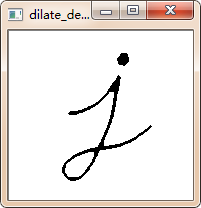# OpenCV+python實現膨脹和腐蝕的示例

### 1，概念及原理：

（1）將圖像 A 與任意形狀的內核 (B)，通常為正方形或圓形，進行卷積。
（2）內核 B 有一個可定義的 錨點, 通常定義為內核中心點。
（3）進行膨脹操作時，將內核 B 劃過圖像A,將內核 B 覆蓋區域的最大相素值提取，並代替錨點位置的相素。顯然，這一最大化操作將會導致圖像中的亮區開始”擴展” (因此有瞭術語膨脹 dilation )。（1）腐蝕在形態學操作傢族裡是膨脹操作的孿生姐妹。它提取的是內核覆蓋下的相素最小值。
（2）進行腐蝕操作時，將內核 B 劃過圖像,將內核 B 覆蓋區域的最小相素值提取，並代替錨點位置的相素。```import cv2 as cv
import numpy as np

def erode_demo(image):
# print(image.shape)
gray = cv.cvtColor(image, cv.COLOR_BGR2GRAY)
ret, binary = cv.threshold(gray, 0, 255, cv.THRESH_BINARY | cv.THRESH_OTSU)
#cv.imshow("binary", binary)
kernel = cv.getStructuringElement(cv.MORPH_RECT, (15, 15))#定義結構元素的形狀和大小
dst = cv.erode(binary, kernel)#腐蝕操作
cv.imshow("erode_demo", dst)

def dilate_demo(image):
#print(image.shape)
gray = cv.cvtColor(image, cv.COLOR_BGR2GRAY)
ret, binary = cv.threshold(gray, 0, 255, cv.THRESH_BINARY | cv.THRESH_OTSU)
#cv.imshow("binary", binary)
kernel = cv.getStructuringElement(cv.MORPH_RECT, (5, 5))#定義結構元素的形狀和大小
dst = cv.dilate(binary, kernel)#膨脹操作
cv.imshow("dilate_demo", dst)

cv.namedWindow("input image", cv.WINDOW_AUTOSIZE)
cv.imshow("input image", src)
erode_demo(src)
dilate_demo(src)

cv.waitKey(0)

cv.destroyAllWindows()```